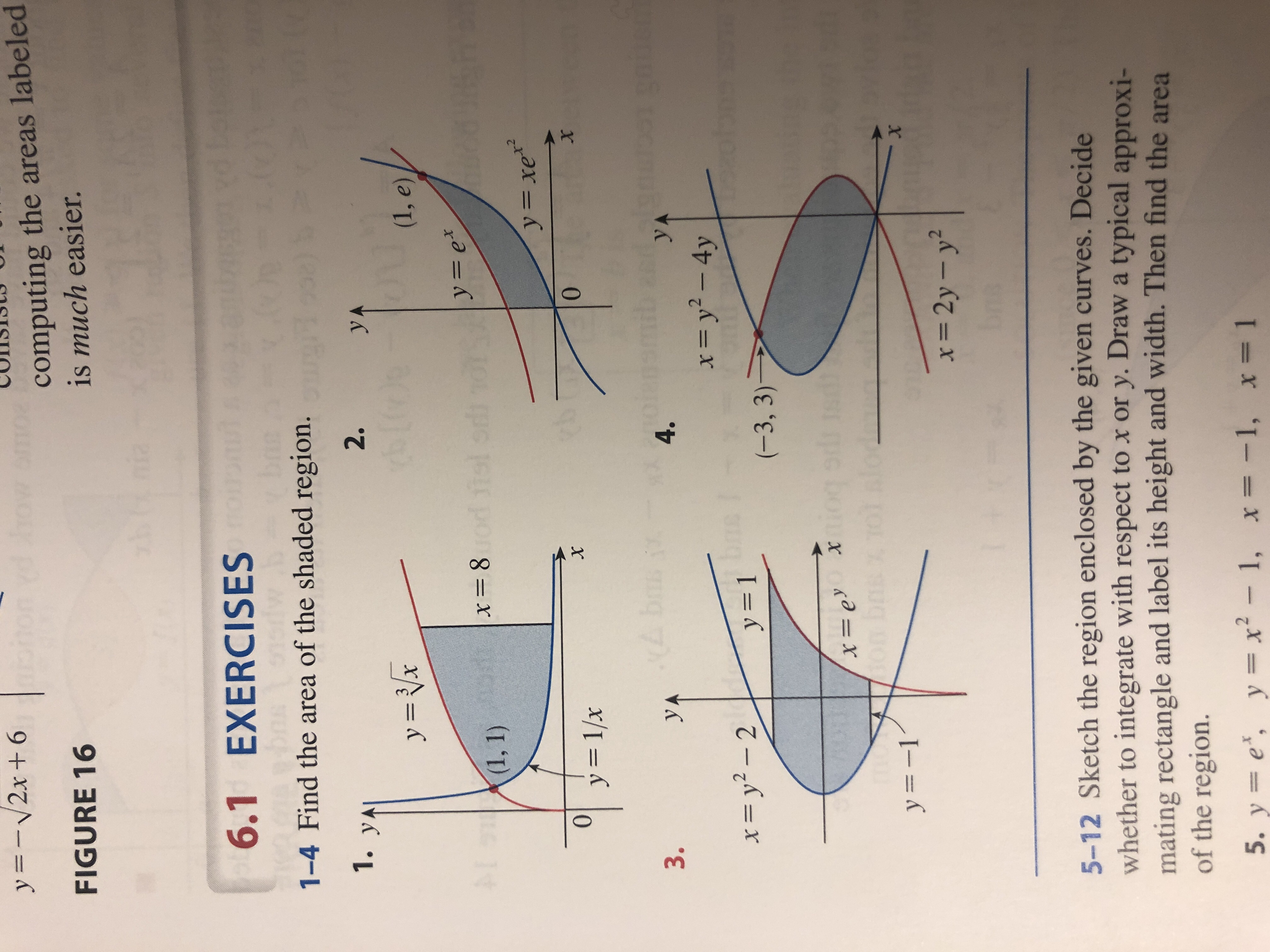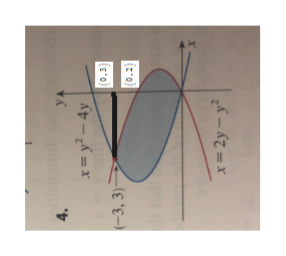y 2x6computing the areas labeledis much easier.FIGURE 165 Hnia6.1 EXERCISES1.1-4 Find the area of the shaded region.1. yA2.y Ay x(1, e)A1D(1, 1)y=exod fl sre-t8yxe0y 1/x0XA bsyA3.4.yA=y2- 4y=y2- 2y 1(-3, 3)Xy=1=2y- y25-12 Sketch the region enclosed by the given curves. Decidewhether to integrate with respect to x or y. Draw a typical approxi-mating rectangle and label its height and width. Then find the areaof the region.5. y e, y = x-1, x= -1, x= 1

Question

#4help_outlineImage Transcriptionclosey 2x6 computing the areas labeled is much easier. FIGURE 16 5 Hnia 6.1 EXERCISES 1. 1-4 Find the area of the shaded region. 1. yA 2. y A y x (1, e) A1 D (1, 1) y=ex od fl sr e-t 8 yxe 0 y 1/x 0 X A bs yA 3. 4. yA =y2- 4y =y2- 2 y 1 (-3, 3) X y=1 =2y- y2 5-12 Sketch the region enclosed by the given curves. Decide whether to integrate with respect to x or y. Draw a typical approxi- mating rectangle and label its height and width. Then find the area of the region. 5. y e, y = x-1, x= -1, x= 1 fullscreen
Step 1

Given,

...help_outlineImage Transcriptionclosex=y - 4y X (0.3) -3, 3) =2y-y2 fullscreen

Want to see the full answer?

See Solution

Want to see this answer and more?

Our solutions are written by experts, many with advanced degrees, and available 24/7

See Solution
Tagged in

Integration